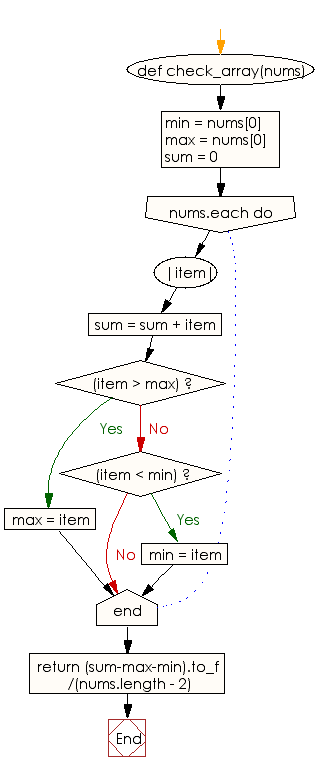﻿ Ruby Array exercises: Compute the average values of a given array of except the largest and smallest values - w3resource# Ruby Array Exercises: Compute the average values of a given array of except the largest and smallest values

## Ruby Array: Exercise-31 with Solution

Write a Ruby program to compute the average values of a given array, except the largest and smallest values. The array length must be 3 or more.

Ruby Code:

``````def check_array(nums)
min = nums
max = nums
sum = 0
nums.each do |item|
sum = sum + item
if(item > max)
max = item
elsif(item < min)
min = item
end
end
return (sum-max-min).to_f/(nums.length - 2)
end

print check_array([3, 4, 5, 6]),"\n"
print check_array([12, 3, 7, 6]),"\n"
print check_array([2, 15, 7, 2]),"\n"
print check_array([2, 15, 7])
``````

Output:

```4.5
6.5
4.5
7.0
```

Flowchart:Ruby Code Editor: